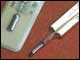Languages:Unit prefixes of the International System of Units (SI)

In order to keep figures short and presentable, SI prefixes are often attached to units such as m (meter) or g (gram).

For example, a substance weighing 0.000 000 001 g may be described more simply as 1 ng (nanogram) or as 1000 pg (picograms).

Common prefixes of the International System of Units (SI) are:

 p pico = 1 000 000 000 000th = 10-12 n nano = 1 000 000 000th = 10-9 µ micro = 1 000 000th = 10-6 m milli = 1 000th = 10-3 no prefix = 1 k kilo = 1 000 = 103 M Mega = 1 000 000 = 106 G Giga = 1 000 000 000 = 109 T Tera = 1 000 000 000 000 = 1012
Translation(s):
Related publications:Mercury
• ABC - DEF - GHI - JKL - MNO - PQRS - TUV - WXYZ
•Get involved!

This summary is free and ad-free, as is all of our content. You can help us remain free and independant as well as to develop new ways to communicate science by becoming a Patron!BECOME A PATRON!

View All